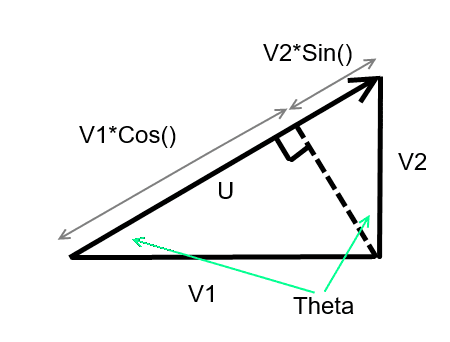# The Magnitude of a Vector

CivilSigma

## Homework Statement

For any vector in 2D space, it can be broken down into its horizontal and vertical components.

## Homework Equations

In one of my engineering classes, we are using the following equation to determine the magnitude of a vector:

$$u=v_1 \cdot cos\theta +u_2 \cdot sin\theta$$

Where $\theta$ is the angle with respect to the horizontal, v1 is the horizontal component and v2 is the vertical component of the vector.

I know this equation works but I don't understand why.
I feel like I am missing a fundamental concept, because to determine the magnitude of a vector, I would use Pythagoras theorem, and I cannot derive the above equation from Pythagoras's equation.

## The Attempt at a Solution

Mentor
Can you provide some context for where this equation is applied? Perhaps give a specific example.

In general, this equation will not work for a single vector whose x and y components are ##u_1## and ##u_2##. Perhaps they are summing the horizontal components of two different vectors to obtain a net horizontal resultant?

Staff Emeritus
Homework Helper
That equation doesn't give the magnitude of the vector. It gives you the component of the vector in the direction of ##\hat n = \cos\theta\,\hat i + \sin\theta\,\hat j##.

Homework Helper
Gold Member
In one of my engineering classes, we are using the following equation to determine the magnitude of a vector:
u=v1⋅cosθ + u2⋅sinθ​

Where $\theta$ is the angle with respect to the horizontal, v1 is the horizontal component and v2 is the vertical component of the vector.

I know this equation works but I don't understand why.

It comes from geometry... See this diagram... If that's not clear do say and I will explain some more.#### Attachments

•CivilSigma
Mentor
I think you meant to write the equation as $$u=u_1\cos{\theta}+u_2\sin{\theta}\tag{1}$$where $$u_1=u\cos{\theta}\tag{2}$$and$$u_2=u\sin{\theta}\tag{3}$$If you substitute Eqns. 2 and 3 into Eqn. 1, you get:
$$u=u\cos^2{\theta}+u\sin^2{\theta}=u$$

•CivilSigma and SammyS